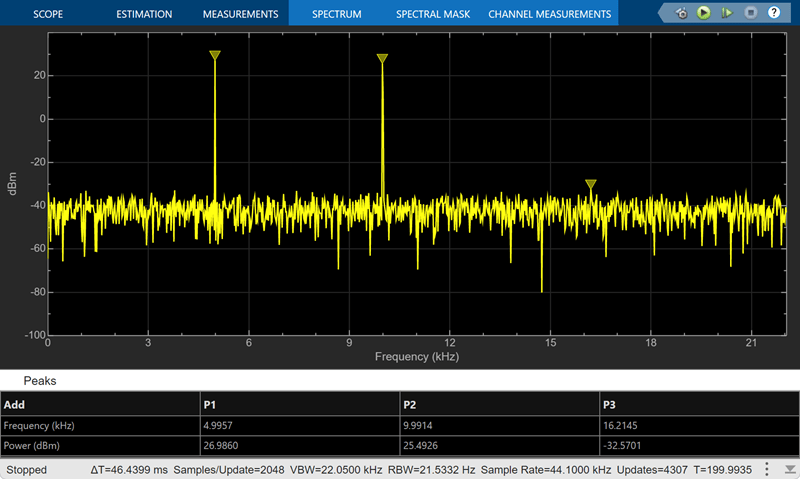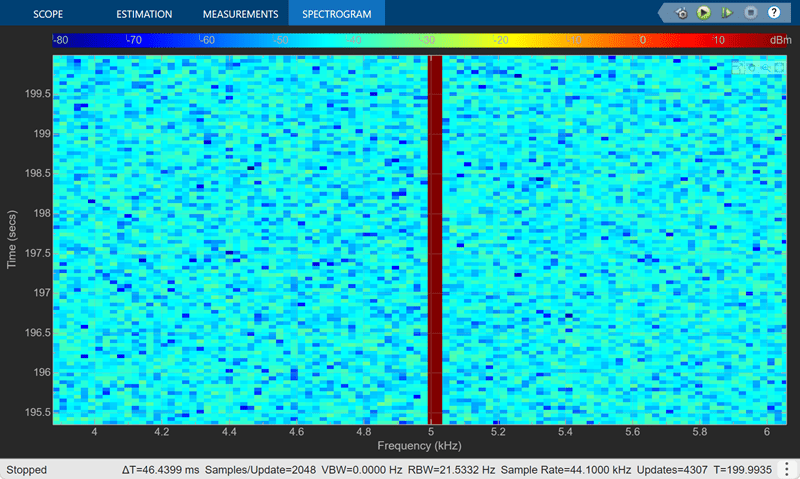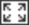# View the Spectrogram Using Spectrum Analyzer

Spectrograms are a two-dimensional representation of the power spectrum of a signal as this signal sweeps through time. They give a visual understanding of the frequency content of your signal. Each line of the spectrogram is one periodogram computed using either the filter bank approach or the Welch's algorithm of averaging modified periodogram.

To show the concepts of the spectrogram, this example uses the `ex_psd_sa` model as the starting point.

Open the model and double-click the Spectrum Analyzer block. In the Analyzer tab, select Spectrogram. In the Estimation tab, Method is set to `Filter bank`. Run the model. You can see the spectrogram output in the spectrum analyzer window. To acquire and store the data for further processing, create a `SpectrumAnalyzerBlockConfiguration` object and run the `getSpectrumData` function on this object.### Colormap

Power spectrum is computed as a function of frequency `f` and is plotted as a horizontal line. Each point on this line is given a specific color based on the value of the power at that particular frequency. The color is chosen based on the colormap seen at the top of the display. To change the colormap, click Analyzer tab > Settings. A Spectrum Analyzer Settings window opens. Under Display and Labels, choose one of the options for Color Map. By default, Color Map is set to `jet`.

The two frequencies of the sine wave are distinctly visible at 5 kHz and 10 kHz. Since the spectrum analyzer uses the filter bank approach, there is no spectral leakage at the peaks. The sine wave is embedded in Gaussian noise, which has a variance of 0.0001. This value corresponds to a power of -40 dBm. The color that maps to -40 dBm is assigned to the noise spectrum. The power of the sine wave is 26.9 dBm at 5 kHz and 10 kHz. The color used in the display at these two frequencies corresponds to 26.9 dBm on the colormap.

To confirm the dBm values, select Spectrum in the Analyzer tab. Enable the peak finder measurements in the Measurements tab. This view shows the power of the signal at various frequencies.You can see that the two peaks in the power display have an amplitude of about 26 dBm and the white noise is averaging around -40 dBm.

### Display

In the spectrogram display, time scrolls from top to bottom, so the most recent data is shown at the top of the display. As the simulation time increases, the offset time also increases to keep the vertical axis limits constant while accounting for the incoming data.

### Resolution Bandwidth (RBW)

Resolution Bandwidth (RBW) is the minimum frequency bandwidth that can be resolved by the spectrum analyzer. By default, RBW (Hz) is set to `Auto`. In the auto mode, RBW is the ratio of the frequency span to 1024. In a two-sided spectrum, this value is Fs/1024, while in a one-sided spectrum, it is (Fs/2)/1024. In this example, RBW is (44100/2)/1024 or 21.53 Hz.

If the Method is set to `Filter bank`, using this value of RBW, the number of input samples used to compute one spectral update is given by, which is 44100/21.53 or 2048 in this example.

If the Method is set to `Welch`, using this value of RBW,is computed iteratively using this relationship:where, Op is the amount of overlap between the previous and current buffered data segments. NENBW is the equivalent noise bandwidth of the window. For more information on the details of the spectral estimation algorithm, see Spectral Analysis.

To distinguish between two frequencies in the display, the distance between the two frequencies must be at least RBW. In this example, the distance between the two peaks is 5000 Hz, which is greater than RBW. Hence, you can see the peaks distinctly.

Change the frequency of the second sine wave from 10000 Hz to 5015 Hz. The difference between the two frequencies is 15 Hz, which is less than RBW.On zooming, you can see that the peaks at 5000 Hz and 5015 Hz are not distinguishable.To increase the frequency resolution, decrease RBW to 1 Hz and run the simulation. On zooming, the two peaks, which are 15 Hz apart, are now distinguishable.### Time Resolution

Time resolution is the distance between two spectral lines in the vertical axis. By default, Time Resolution (s) in the Spectrogram tab is set to `Auto`. In this mode, the value of time resolution is 1/RBW s, which is the minimum attainable resolution. When you increase the frequency resolution, the time resolution decreases. To maintain a good balance between the frequency resolution and time resolution, change the RBW (Hz) to `Auto`. You can also specify the Time Resolution (s) as a numeric value.

### Scale Color Limits

When you run the model and do not see the spectrogram colors, click the autoscale button shown below. This option autoscales the colors.The spectrogram updates in real time. During simulation, if you change any of the tunable parameters in the model, the changes are effective immediately in the spectrogram.